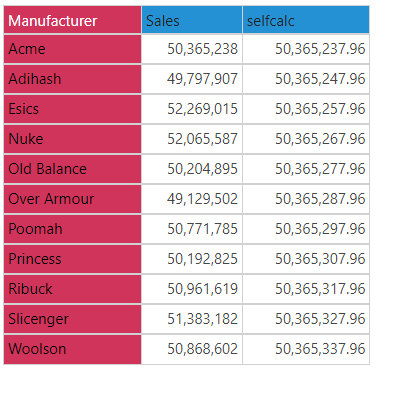# SelfCalc

The SelfCalc function is used to perform recursive calculations. Recursive calculations are used to perform a series of calculations in the query, based on a given current member.

• Returned Output: Variant
• Version: 2020.15
• Compatibility: SAP BW and Microsoft MDX Engines

#### Syntax & Usage

.selfcalc

• In MDX, recursive calculations are constructed by adding the current calculation ID inside the actual calculation.
• In Pyramid, recursive calculations can be constructed by adding the 'selfcalc' function to the expression.
• Pyramid will convert the 'selfcalc' argument into MDX syntax by translating it into the current calculation id, producing a recursive calculation.
##### Example. If Statement

In this example, an If statement is constructed. The calculation should return sales for the current member; if the item returned is not the current member, then it should return sales for the previous member:

iif([dim].[att].currentmember.name = ‘item name’, [measures].[sales], ([dim].[att].prevmember, [measures].selfcalc))

Pyramid will translate the expression into the following MDX calculation, where the 'selfcalc' argument is replaced by the current calculation ID:

member [measures].[+1234-abcd-5678-ef00+] as iif([dim].[att].currentmember.name = ‘item name’, [measures].[sales], ([dim].[att].prevmember, [measures].[+1234-abcd-5678-ef00+]))

Taking this example, the currentmember function is used to inject the manufacturer 'Acme' as the criteria for the If statement. If the statement resolves to true, sales for Acme will be returned. If that statement resolves to false, sales for the previous member in the Manufacturer hierarchy will be returned, plus 10:

iif([Manfucaturer].[Manufacturer].currentmember is [Manfucaturer].[Manufacturer].&, [Measures].[Sales], ([Measures].selfcalc, [Manfucaturer].[Manufacturer].prevmember) + 10)

In the resulting grid, the first row is Acme; this row shows the sales for Acme. All subsequent rows do not contain Acme, so they return sales for the previous member + 10. For instance, the row for Esics shows the sales for Adishash + 10: